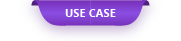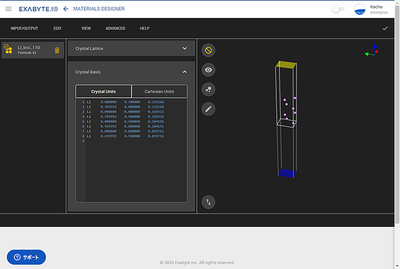Here, we will introduce how to calculate the work function of metal crystals.

# Electron Work Function using the ESM method

Here, we will introduce how to calculate the work function of metal crystals.

1. Optimization of crystal structure
2. Slab model creation
3. ESM model creation
4. Structural optimization to which the ESM method is applied
5. Work function value comparison

#### 1. Crystal structure optimization

First of all, the calculation is performed with Li of the BCC structure obtained from Material Projects. I used Quantum ESPRESSO as the calculation software.

Table 1 Lattice constants of Materials Projects and Quantum ESPRESSO

#### 2. Slab model creation

Create a metal slab model based on the optimized crystal structure. It is also possible to create using the slab model installed in Exabyte.io or NanoLabo.

Here, 100 faces, 110 faces, and 111 faces were created.

* NanoLabo can also be used on Exabyte.io.

#### 3. ESM model creation

By using the ESM method function of Quantum ESPRESSO, it is possible to obtain accurately without taking a lot of vacuum layers. Therefore, here, we will create a model for calculation using the ESM method function of Quantum ESPRESSO. The model is created from "Boundary Conditions". This time, I chose bc3 as the Type.

Save the ESM model you created.

#### 4. Structural optimization adapted to the ESM method

Exabyte.io provides a computational workflow that uses the ESM method. This time, we will use “Effective Screening Medium (ESM) Relax”.

#### 5. Work function value comparison

The calculation result is displayed as below.

By using the ESM method, the potential at the end becomes a value of zero, so the value with the sign of the Fermi level changed becomes the work.